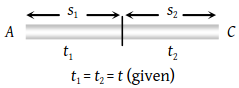A body is moving with uniform acceleration describes 40 m in the first 5 sec and 65 m in next 5 sec. Its initial velocity will be

(1) 4 m/s

(2) 2.5 m/s

(3) 5.5 m/s

(4) 11 m/s

Concept Videos :-

#26-Equations-for-Uniform-Acceleration
#27-Equations for Uniform Acceleration 28Calculus Method29
#28-Sign Convention
#29-Solved Examples 16
#31-Solved-Examples-18
#32-Solved Examples 19
#33-Solved Examples 20
#34-Solved-Examples-21
#35 Solved Examples 22
#30-Solved Examples 17

Concept Questions :-

Uniformly accelerated motion

(3) $\because {S}_{1}=ut+\frac{1}{2}a{t}^{2}$   .....(i)

and velocity after first t sec

$v=u+at$Now, ${S}_{2}=vt+\frac{1}{2}a{t}^{2}$

$=\left(u+at\right)t+\frac{1}{2}a{t}^{2}$  ..... (ii)

Equation (ii) – (i) $⇒{S}_{2}-{S}_{1}=a{t}^{2}$

$⇒a=\frac{{S}_{2}-{S}_{1}}{{t}^{2}}=\frac{65-40}{{\left(5\right)}^{2}}=1\text{\hspace{0.17em}}m/{s}^{2}$

From equation (i), we get,

${S}_{1}=ut+\frac{1}{2}a{t}^{2}⇒40=5u+\frac{1}{2}×1×25$

Difficulty Level:

• 8%
• 19%
• 64%
• 11%